Browse by Topic

# Embracing Uncertainty: Better Model Selection with Bayesian Linear Regression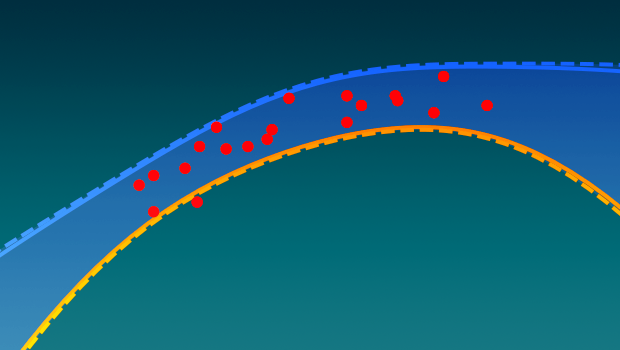Readers who follow the Mathematica Stack Exchange (which I highly recommend to any Wolfram Language user) may have seen this post recently, in which I showed a function I wrote to make Bayesian linear regression easy to do. After finishing that function, I have been playing around with it to get a better feel of what it can do, and how it compares against regular fitting algorithms such as those used by Fit. In this blog post, I don’t want to focus too much on the underlying technicalities (check out my previous blog post to learn more about Bayesian neural network regression); rather, I will show you some of the practical applications and interpretations of Bayesian regression, and share some of the surprising results you can get from it.

## Getting Started

The easiest way to get my BayesianLinearRegression function is through my submission to the Wolfram Function Repository. To use this version of the function with the code examples in this blog, you can evaluate the following line, which creates a shortcut for the resource function: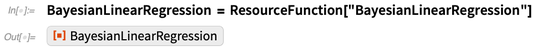✕ `BayesianLinearRegression = ResourceFunction["BayesianLinearRegression"]`

You can also visit the GitHub repository and follow the installation instructions to load the BayesianInference package with the following: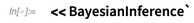✕ `<< BayesianInference``

Alternatively, you can get the standalone source file of BayesianLinearRegression and evaluate it to make the function available to you, though the function regressionPlot1D I use later will not work in the following examples if you don’t get the full BayesianInference package. Its definition is in the BayesianVisualisations.wl file.

## Back to Basics

I want to do something that will be very familiar to most people with some background in data fitting: polynomial regression. I could have picked something more complicated, but it turns out that the Bayesian take on fitting data adds quite a bit of depth and new possibilities even to something as simple as fitting polynomials, making it an ideal demonstration example.

I picked the example data here specifically because it hints at a straight trend, while still leaving some doubt: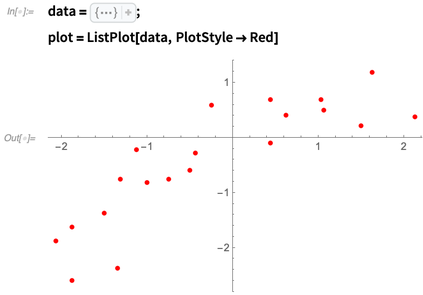✕ ```data = CompressedData[" 1:eJxTTMoPSmViYGAQAWIQDQE/9kPobxC64SuUz3wAKm8Poc9A6IYPUP4zKP0A qv4xlP4HoQ+wQPX/gqr7DNXPcABFHcNHmDlQ+g5U/BKUfoFGMzpA6Bsw9Wju +Yqm/hOUPgOln0DV3YLSF2Dut0d11y8o/QFKv9qPat+O/QBd7zpq "]; plot = ListPlot[data, PlotStyle -> Red]```

So let’s start off by doing the first sensible thing to do: fit a line through it. Concretely, I will fit the model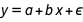, where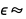NormalDistribution[0,σ]. BayesianLinearRegression uses the same syntax as LinearModelFit, but it returns an association containing all relevant information about the fit: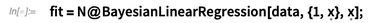✕ `fit = N@BayesianLinearRegression[data, {1, \[FormalX]}, \[FormalX]];`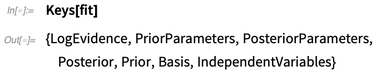✕ `Keys[fit]`

I will focus most on explaining the posterior distributions and the log-evidence. The log-evidence is a number that indicates how well the model fits with the data: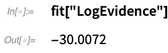✕ `fit["LogEvidence"]`

Is this value good or bad? We can’t tell yet: it only means something when compared to the log-evidences of other fits of the same data. I will come back to model comparison in the section on the Bayesian Occam’s razor, but let’s first take a look in more detail at the linear fit we just computed. The fit association has a key called "Posterior" that contains a number of useful probability distributions: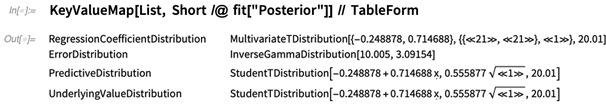✕ `KeyValueMap[List, Short /@ fit["Posterior"]] // TableForm`

In Bayesian inference, the word “posterior” refers to a state of knowledge after having observed the data (as opposed to “prior,” which refers to the state where you do not know the data yet). The posterior distribution of the regression parameters tells us how well the parameters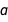and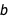are constrained by the data. It’s best visualized with a ContourPlot: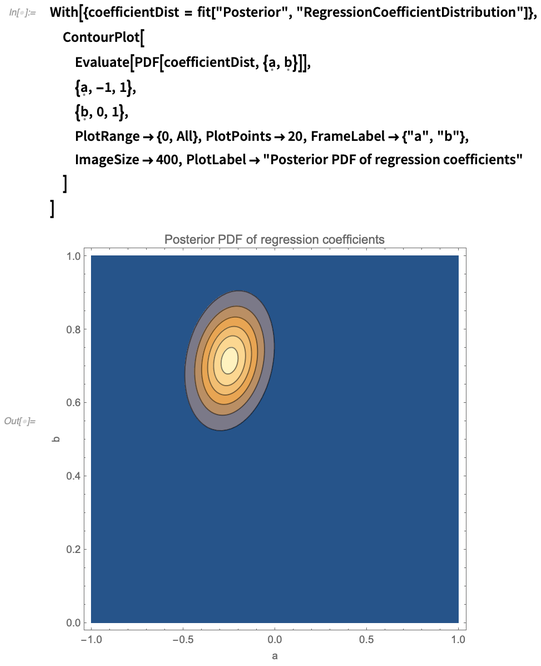✕ ```With[{coefficientDist = fit["Posterior", "RegressionCoefficientDistribution"]}, ContourPlot[ Evaluate[PDF[coefficientDist, {\[FormalA], \[FormalB]}]], {\[FormalA], -1, 1}, {\[FormalB], 0, 1}, PlotRange -> {0, All}, PlotPoints -> 20, FrameLabel -> {"a", "b"}, ImageSize -> 400, PlotLabel -> "Posterior PDF of regression coefficients" ] ]```

The slant of the ellipses in the contour plot shows that there seems to be some positive correlation between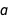and. You can calculate exactly how much correlation there is between them with Correlation: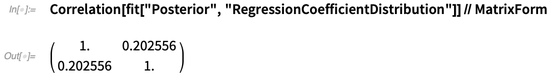✕ ```Correlation[ fit["Posterior", "RegressionCoefficientDistribution"]] // MatrixForm```

At first glance, this may seem strange. Why areandcorrelated with each other? One way to look at this is to consider how the fit changes when you force one of the two coefficients to change. For example, you can fixand then try and find the best value ofthat fits the data with FindFit. The following illustrates this thought experiment: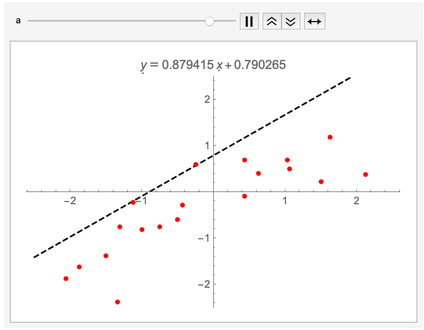✕ ```With[{dat = data, p = plot}, Animate[ With[{fit = a + \[FormalB] \[FormalX] /. FindFit[dat, a + \[FormalB] \[FormalX], \[FormalB], \[FormalX]]}, Show[ Plot[ fit, {\[FormalX], -2.5, 2.5}, PlotLabel -> \[FormalY] == fit, PlotRange -> {-2.5, 2.5}, PlotStyle -> Directive[Black, Dashed] ], p ] ], {{a, 0.}, -1, 1}, TrackedSymbols :> {a}, AnimationDirection -> ForwardBackward ] ]```

Intuitively, there is correlation between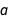and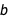because the center of mass of the points is slightly to the bottom left of the origin: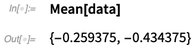✕ `Mean[data]`

This means that whenincreases,also needs to increase so that it can still catch the center of mass of the cloud. Here I’ve moved all points even further down and left to amplify the effect: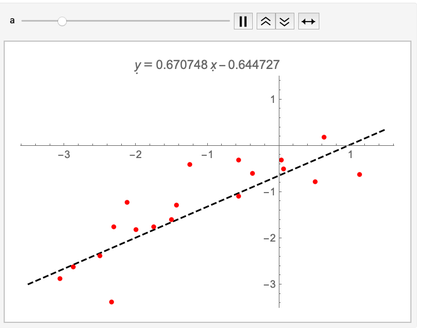✕ ```With[{dat = data - 1}, Animate[ With[{fit = a + \[FormalB] \[FormalX] /. FindFit[dat, a + \[FormalB] \[FormalX], \[FormalB], \[FormalX]]}, Show[ Plot[ fit, {\[FormalX], -3.5, 1.5}, PlotLabel -> \[FormalY] == fit, PlotRange -> {-3.5, 1.5}, PlotStyle -> Directive[Black, Dashed] ], ListPlot[dat, PlotStyle -> Red] ] ], {{a, 0.}, -1, 1}, TrackedSymbols :> {a}, AnimationDirection -> ForwardBackward ] ]```

Another way to think of the posterior distribution overandis to think of the fit as consisting of infinitely many lines, drawn at random across the page and with each line weighted according to how well it fits the data. Calculating that weight is essentially what Bayesian inference is about. In the following plot, I used RandomVariate to draw many lines from the posterior. By making the opacity of each individual line quite low, you can see the regions where the lines tend to concentrate: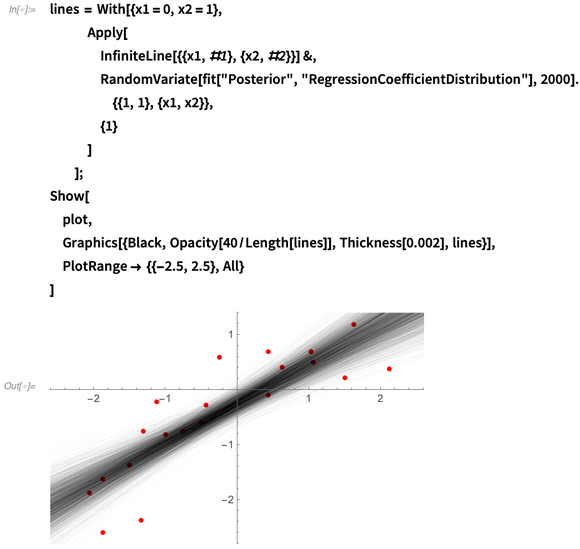✕ ```lines = With[{x1 = 0, x2 = 1}, Apply[ InfiniteLine[{{x1, #1}, {x2, #2}}] &, RandomVariate[ fit["Posterior", "RegressionCoefficientDistribution"], 2000].{{1, 1}, {x1, x2}}, {1} ] ]; Show[ plot, Graphics[{Black, Opacity[40/Length[lines]], Thickness[0.002], lines}], PlotRange -> {{-2.5, 2.5}, All} ]```

The distribution of this cloud of lines is what I like to call the “underlying value distribution,” which is one of the distributions returned by the fit. To plot it, it’s convenient to use InverseCDF to calculate quantiles of the distribution. In the next example, I plotted the 5-, 50- and 95-percent quantiles, meaning that you’d expect 90% of the lines to fall within the shaded areas: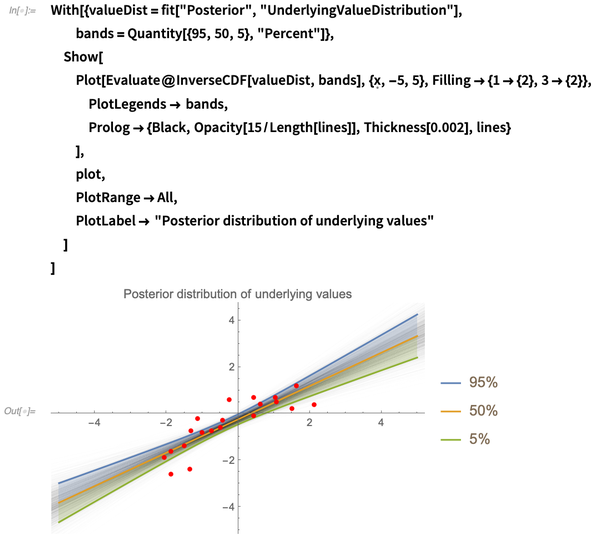✕ ```With[{valueDist = fit["Posterior", "UnderlyingValueDistribution"], bands = Quantity[{95, 50, 5}, "Percent"]}, Show[ Plot[Evaluate@InverseCDF[valueDist, bands], {\[FormalX], -5, 5}, Filling -> {1 -> {2}, 3 -> {2}}, PlotLegends -> bands, Prolog -> {Black, Opacity[15/Length[lines]], Thickness[0.002], lines} ], plot, PlotRange -> All, PlotLabel -> "Posterior distribution of underlying values" ] ]```

BayesianLinearRegression also estimates the standard deviation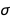of the error term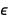, and just like the regression coefficientsand, it has its own distribution. Variance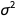follows an InverseGammaDistribution, so to get the distribution of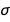I use TransformedDistribution: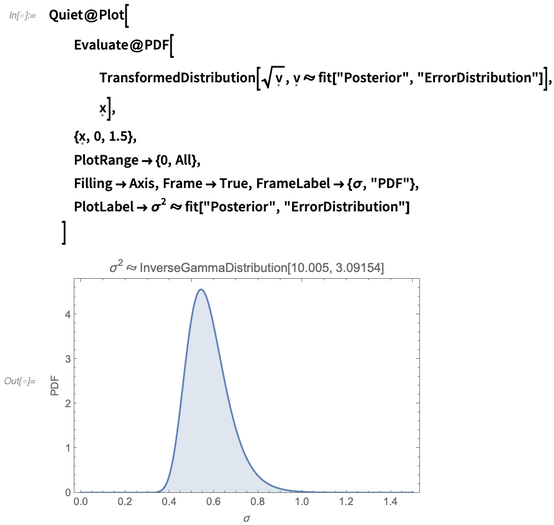✕ ```Quiet@Plot[ Evaluate@PDF[ TransformedDistribution[ Sqrt[\[FormalV]], \[FormalV] \[Distributed] fit["Posterior", "ErrorDistribution"]], \[FormalX]], {\[FormalX], 0, 1.5}, PlotRange -> {0, All}, Filling -> Axis, Frame -> True, FrameLabel -> {\[Sigma], "PDF"}, PlotLabel -> \[Sigma]^2 \[Distributed] fit["Posterior", "ErrorDistribution"] ]```

So in short, the posterior error terms are distributed asNormalDistribution[0, σ], where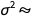InverseGammaDistribution[10.005,3.09154]. This distribution we can calculate with ParameterMixtureDistribution: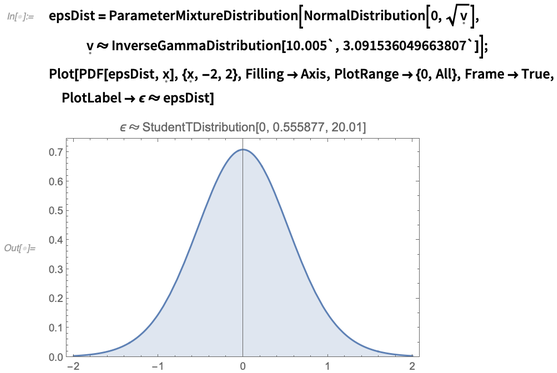✕ ```epsDist = ParameterMixtureDistribution[ NormalDistribution[0, Sqrt[\[FormalV]]], \[FormalV] \[Distributed] InverseGammaDistribution[10.005`, 3.091536049663807`]]; Plot[PDF[epsDist, \[FormalX]], {\[FormalX], -2, 2}, Filling -> Axis, PlotRange -> {0, All}, Frame -> True, PlotLabel -> \[Epsilon] \[Distributed] epsDist]```

## Uncertain Uncertainties

Just to make the point clear: there is uncertainty about the uncertainty of the model, which for me was an idea that was a bit difficult to get my head around initially. To make things even more complicated, all of the lines I mentioned previously also have distributions of error bars that correlate withand. So if you want to make a prediction from this model, you need to consider an infinite number of trend lines that each carry an infinite number of error bars. That’s a lot of uncertainty to consider!

Thinking about regression problems this way makes it clear why Bayesian inference can be a daunting task that involves lots of complicated integrals. For linear regression, though, we’re fortunate enough that it’s possible to do all of these integrals symbolically and plow our way through the infinities. This is one of the reasons why scientists and engineers like linear mathematics so much: everything remains tractable, with nice-looking formulas.

The distribution that tells you where to expect to find future data (taking all of the aforementioned uncertainties into consideration) is called the posterior predictive distribution. For the data I just fitted, it looks like this: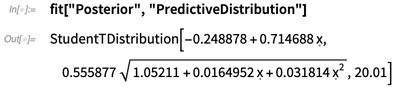✕ `fit["Posterior", "PredictiveDistribution"]`

Now I will plot this distribution using the function regressionPlot1D from the Git repository, which is just shorthand for the underlying values plot I showed earlier. I also included the distribution you get when you make a “point estimate” of the predictive model. This means that you take the best values of,andfrom the posterior and plotusing those values as if they were completely certain. Point estimates can be useful in making your results more manageable since probability distributions can be difficult to work with, but reducing your distributions to single numbers always discards information. Here I used the Mean (expected value) to reduce the distributions to single values, but you could also use other measures of centrality like the median or mode: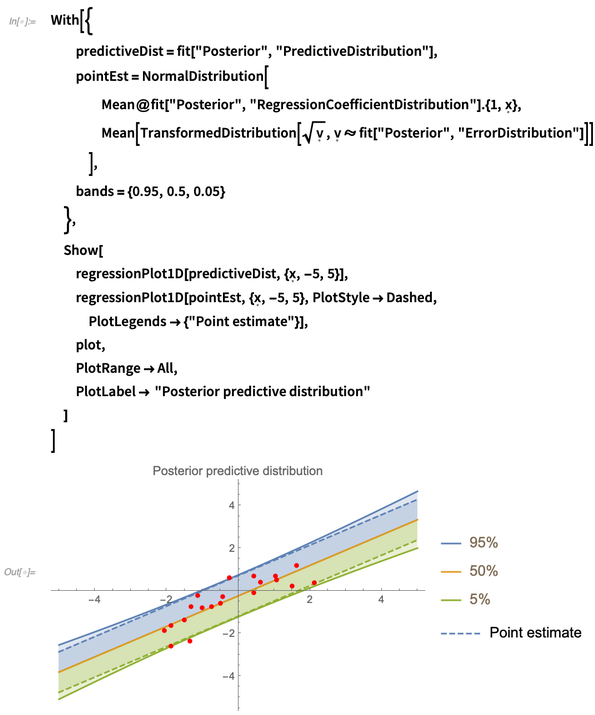✕ ```With[{ predictiveDist = fit["Posterior", "PredictiveDistribution"], pointEst = NormalDistribution[ Mean@fit["Posterior", "RegressionCoefficientDistribution"].{1, \[FormalX]}, Mean[TransformedDistribution[ Sqrt[\[FormalV]], \[FormalV] \[Distributed] fit["Posterior", "ErrorDistribution"]]] ], bands = {0.95, 0.5, 0.05} }, Show[ regressionPlot1D[predictiveDist, {\[FormalX], -5, 5}], regressionPlot1D[pointEst, {\[FormalX], -5, 5}, PlotStyle -> Dashed, PlotLegends -> {"Point estimate"}], plot, PlotRange -> All, PlotLabel -> "Posterior predictive distribution" ] ]```

So that covers fitting a straight line through the points. However, the shape of the data suggests that maybe a quadratic fit is more appropriate (I cooked up the example just for this purpose, of course), so this is a good moment to demonstrate how Bayesian model comparison works. I am going to fit polynomials up to degree 5 to the data, with each of these polynomials representing a model that could explain the data. From this micro-universe of possible explanations of the data, I want to select the best one.

## The Bayesian Occam’s Razor

In Bayesian inference, the models have probability distributions in the same way that the regression coefficients,andhave distributions. The posterior probability of a model depends on two factors:

• A quantity commonly referred to as evidence or marginal likelihood. This measures how well a model fits the data while taking into account the uncertainties in the regression parameters such as,and. BayesianLinearRegression reports this quantity as the "LogEvidence": the higher the log-evidence, the better the model. The evidence automatically accounts for model complexity due to an effect that is sometimes referred to as the Bayesian Occam’s razor (see, for example, chapter 28 in this book by David MacKay).
• The prior probability of the model.

Usually you’ll only want to consider models that, before seeing the data, you consider approximately equally likely. However, sometimes you do have strong prior information available that the data was generated by a certain model, and you’d only accept other explanations if the evidence overwhelmingly disproves that model in favor of another.

For example, if the data shown previously came from a noisy (and possibly biased) measurement of current through a standard resistor as a function of voltage, I would have little doubt that Ohm’s law applies here and that fitting a straight line is the correct way to interpret the data. It’s simply more likely that a little noise accidentally made the data look quadratic rather than that Ohm’s law suddenly stopped working in my experiment. In this case, I would assign a prior probability very close to 1 to Ohm’s law and divide the remaining sliver of probability among competing models I’m willing to consider. This is in accordance with a principle called Cromwell’s rule, which was coined by Dennis Lindley:

“Leave a little probability for the Moon being made of green cheese; it can be as small as 1 in a million, but have it there since otherwise an army of astronauts returning with samples of the said cheese will leave you unmoved.”

So let’s see how the fits of different polynomials look and what their evidence is: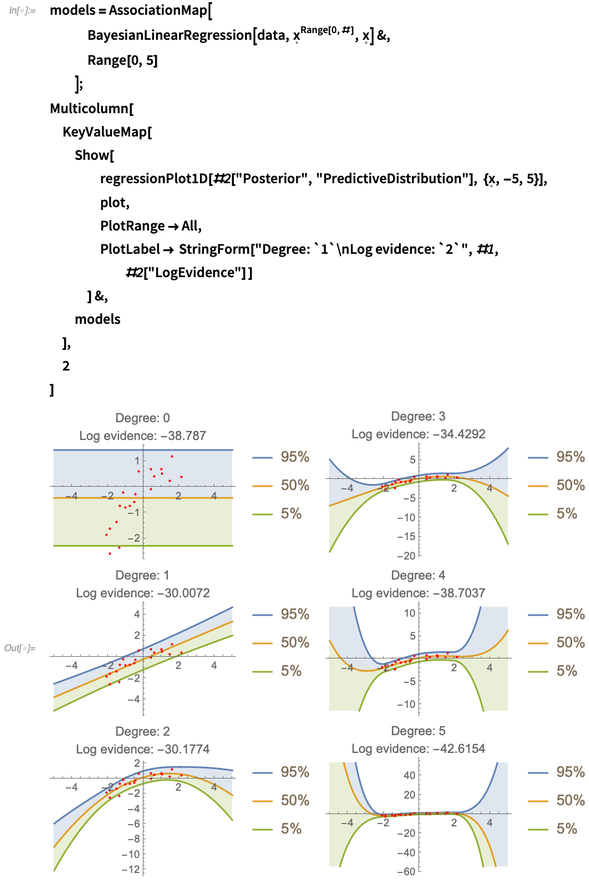✕ ```models = AssociationMap[ BayesianLinearRegression[data, \[FormalX]^ Range[0, #], \[FormalX]] &, Range[0, 5] ]; Multicolumn[ KeyValueMap[ Show[ regressionPlot1D[#2["Posterior", "PredictiveDistribution"], {\[FormalX], -5, 5}], plot, PlotRange -> All, PlotLabel -> StringForm[ "Degree: `1`\nLog evidence: `2`", #1, #2["LogEvidence"] ] ] &, models ], 2 ]```

As you can see, the first- and second-degree fits are in close competition for being the best model. If we assume that the prior probabilities for all models are the same, we can calculate the relative probabilities of the fits by exponentiating the log-evidences and normalizing them. For this operation, we can borrow SoftmaxLayer from the neural network framework: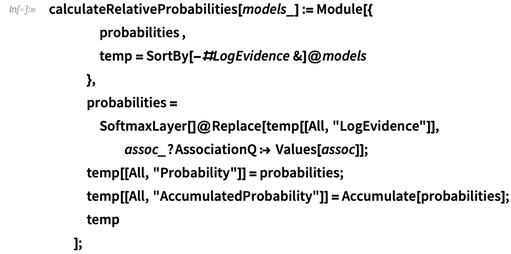✕ ```calculateRelativeProbabilities[models_] := Module[{ probabilities , temp = SortBy[-#LogEvidence &]@models }, probabilities = SoftmaxLayer[]@ Replace[temp[[All, "LogEvidence"]], assoc_?AssociationQ :> Values[assoc]]; temp[[All, "Probability"]] = probabilities; temp[[All, "AccumulatedProbability"]] = Accumulate[probabilities]; temp ];```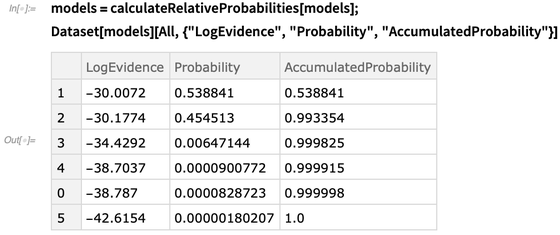✕ ```models = calculateRelativeProbabilities[models]; Dataset[models][All, {"LogEvidence", "Probability", "AccumulatedProbability"}]```

In this table, I sorted the models by probabilities and then accumulated them so you can easily see how much total probability you’ve covered going from top to bottom. Keep in mind that these probabilities only mean anything in the micro-universe we’re currently looking at: the moment I would start adding more models, all of these probabilities could change. In Bayesian inference, you can only compare models you’re willing to formulate in the first place: there is no universal measure that tells you if the fit of the data is actually good or not in some completely objective sense. All you can really hope for is to find the best model(s) from the group you’re considering because the set of models you’re not considering is impossible to compare against.

### Interlude: Comparing against LinearModelFit

At this point, it’s also good to compare the fits by BayesianLinearRegression against those by LinearModelFit, which also presents several goodness-of-fit measures: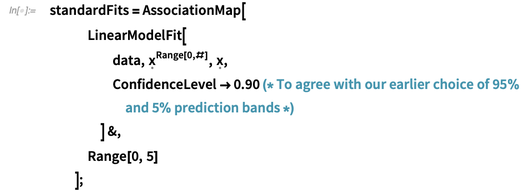✕ ```standardFits = AssociationMap[ LinearModelFit[ data, \[FormalX]^Range[0, #], \[FormalX], ConfidenceLevel -> 0.90 (* To agree with our earlier choice of 95% and 5% prediction bands *) \ ] &, Range[0, 5] ];```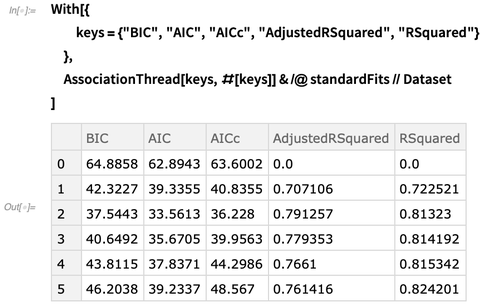✕ ```With[{ keys = {"BIC", "AIC", "AICc", "AdjustedRSquared", "RSquared"} }, AssociationThread[keys, #[keys]] & /@ standardFits // Dataset ]```

As expected, the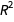measure goes up with the degree of the model since it does not penalize for model complexity. All the other measures seem to favor the second-order model: keep in mind that better models have lower values for the Bayesian information criterion (or BIC, which is an approximation to the negative log-evidence), Akaike information criterion (AIC) and AIC corrected (for small sample size). For adjusted, the highest value indicates the best model. Let’s also compare the prediction bands from LinearModelFit with the Bayesian ones for the second-order model: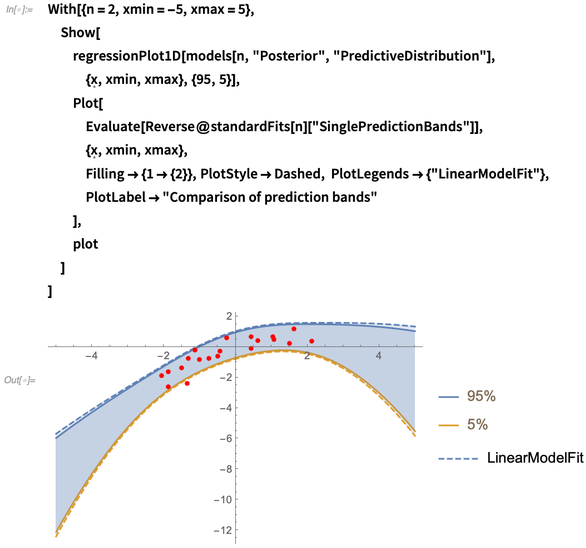✕ ```With[{n = 2, xmin = -5, xmax = 5}, Show[ regressionPlot1D[ models[n, "Posterior", "PredictiveDistribution"], {\[FormalX], xmin, xmax}, {95, 5}], Plot[ Evaluate[Reverse@standardFits[n]["SinglePredictionBands"]], {\[FormalX], xmin, xmax}, Filling -> {1 -> {2}}, PlotStyle -> Dashed, PlotLegends -> {"LinearModelFit"}, PlotLabel -> "Comparison of prediction bands" ], plot ] ]```

As you can see, the confidence bands from LinearModelFit are slightly wider (and therefore more pessimistic) than the posterior prediction bands. The main reason for this difference is that the Bayesian prediction bands take into account all correlations in the posterior distribution between the model parameters and propagates these correlations into the predictions to narrow them down a little bit further. Another way to put it is that the Bayesian analysis does not discard information prematurely when calculating the prediction bands because it retains all intermediate distributions fully. This effect becomes more pronounced when less data is available, as the following fits illustrate (if you’re skeptical about the role of the Bayesian prior in this example, I recommend you try this for yourself with decreasingly informative priors):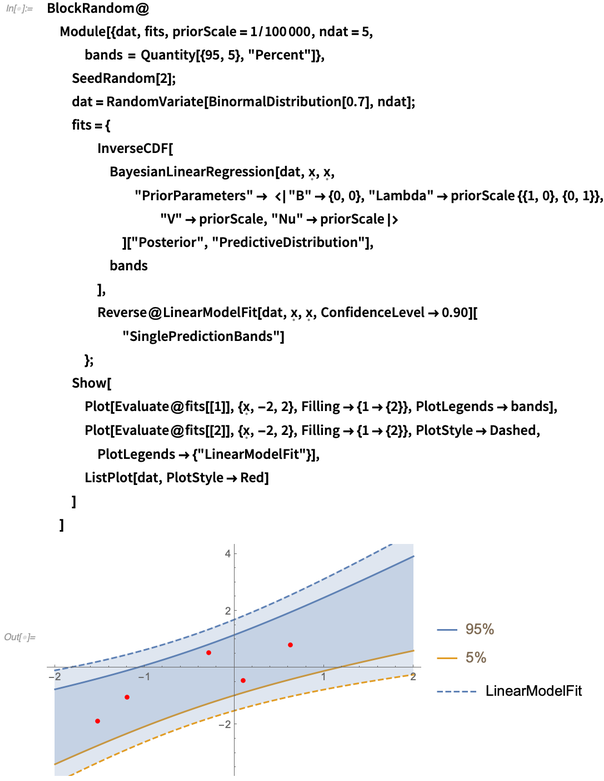✕ ```BlockRandom@ Module[{dat, fits, priorScale = 1/100000, ndat = 5, bands = Quantity[{95, 5}, "Percent"]}, SeedRandom; dat = RandomVariate[BinormalDistribution[0.7], ndat]; fits = { InverseCDF[ BayesianLinearRegression[dat, \[FormalX], \[FormalX], "PriorParameters" -> <|"B" -> {0, 0}, "Lambda" -> priorScale {{1, 0}, {0, 1}}, "V" -> priorScale, "Nu" -> priorScale|> ]["Posterior", "PredictiveDistribution"], bands ], Reverse@ LinearModelFit[dat, \[FormalX], \[FormalX], ConfidenceLevel -> 0.90]["SinglePredictionBands"] }; Show[ Plot[Evaluate@fits[], {\[FormalX], -2, 2}, Filling -> {1 -> {2}}, PlotLegends -> bands], Plot[Evaluate@fits[], {\[FormalX], -2, 2}, Filling -> {1 -> {2}}, PlotStyle -> Dashed, PlotLegends -> {"LinearModelFit"}], ListPlot[dat, PlotStyle -> Red] ] ]```

## Mixing It Up

So now we’re in a position where we have several polynomial models, with two of them competing for the first position and no very clear winner. Which one do we choose? The Bayesian answer to this question is simple: why not both? Why not all? We’re still working from a probabilistic perspective: the truth is simply somewhere in the middle, and there’s no need to make a definite choice. Picking a model is also a form of point estimate, and we’ve seen before that point estimates discard potentially useful information. Instead, we can just split the difference in the same way that we did earlier, by averaging out over all possible values of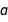,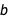and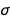while fitting a straight line to the data. The function MixtureDistribution is of great use here to combine the different posterior predictions into a new distribution. It is not even necessary to consider only the first- and second-order models: we can just combine all of them by weight: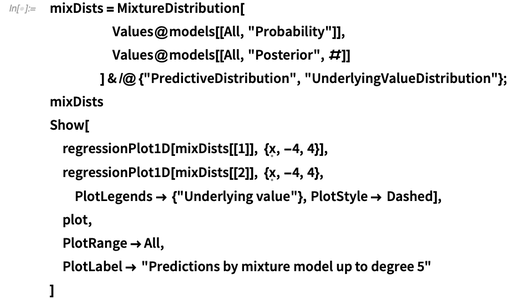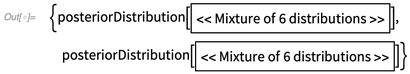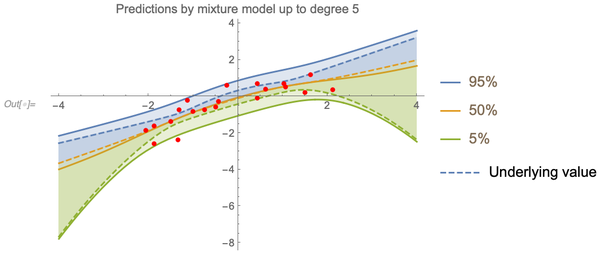✕ ```mixDists = MixtureDistribution[ Values@models[[All, "Probability"]], Values@models[[All, "Posterior", #]] ] & /@ {"PredictiveDistribution", "UnderlyingValueDistribution"}; mixDists Show[ regressionPlot1D[mixDists[], {\[FormalX], -4, 4}], regressionPlot1D[mixDists[], {\[FormalX], -4, 4}, PlotLegends -> {"Underlying value"}, PlotStyle -> Dashed], plot, PlotRange -> All, PlotLabel -> "Predictions by mixture model up to degree 5" ]```

(Note the custom compact formatting of MixtureDistribution from the BayesianInference package.) The underlying value bands highlight the hybridization of models particularly well.

And why even stop there? There are more models we could explore in the polynomial universe, so let’s expand the horizons a bit. For example, why not try a fit like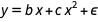(i.e. without the constant offset)? There are 63 different polynomials of up to order 5 to try: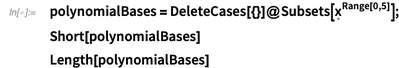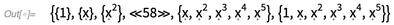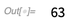✕ ```polynomialBases = DeleteCases[{}]@Subsets[\[FormalX]^Range[0, 5]]; Short[polynomialBases] Length[polynomialBases]```

Here is a function that makes fitting these models a little easier and faster: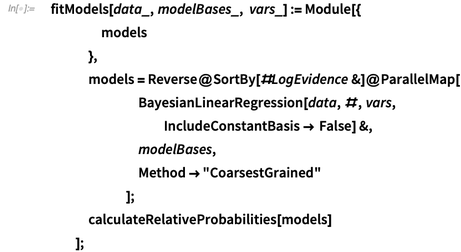✕ ```fitModels[data_, modelBases_, vars_] := Module[{ models }, models = Reverse@SortBy[#LogEvidence &]@ParallelMap[ BayesianLinearRegression[data, #, vars, IncludeConstantBasis -> False] &, modelBases, Method -> "CoarsestGrained" ]; calculateRelativeProbabilities[models] ];```

Fit all possible polynomials up to degree 5, and view the 10 best fits: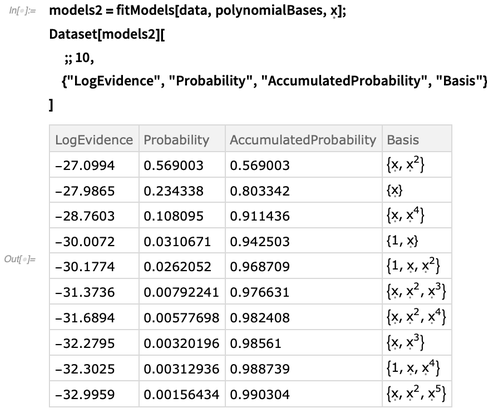✕ ```models2 = fitModels[data, polynomialBases, \[FormalX]]; Dataset[models2][ ;; 10, {"LogEvidence", "Probability", "AccumulatedProbability", "Basis"} ]```

I can now calculate the prediction bands again, but it’s not really necessary to include all models in the mixture since only a few carry any significant weight. To make the distribution more manageable, I will throw away the least likely models that together account for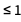percent of the total probability mass: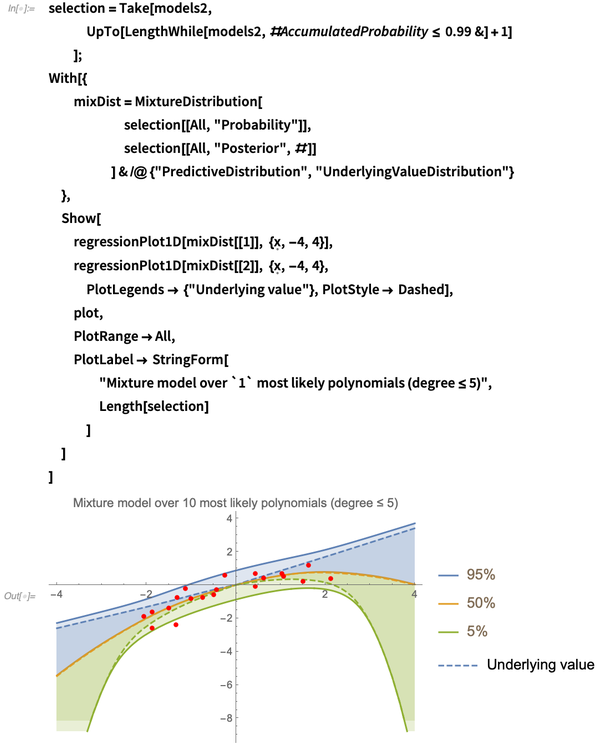✕ ```selection = Take[models2, UpTo[LengthWhile[models2, #AccumulatedProbability <= 0.99 &] + 1] ]; With[{ mixDist = MixtureDistribution[ selection[[All, "Probability"]], selection[[All, "Posterior", #]] ] & /@ {"PredictiveDistribution", "UnderlyingValueDistribution"} }, Show[ regressionPlot1D[mixDist[], {\[FormalX], -4, 4}], regressionPlot1D[mixDist[], {\[FormalX], -4, 4}, PlotLegends -> {"Underlying value"}, PlotStyle -> Dashed], plot, PlotRange -> All, PlotLabel -> StringForm[ "Mixture model over `1` most likely polynomials (degree \ \[LessEqual] 5)", Length[selection] ] ] ]```

It is interesting to see that models with no constant offset are strongly preferred, leading to a very narrow estimate of the underlying value near the origin. The extrapolation bands have also become wider than before, but that’s just a warning about the dangers of extrapolation: if we really have the prior belief that all of these polynomials are equal contenders for explaining the data, then the wide extrapolation bands are a natural consequence of that assumption since there are so many different possible explanations of the data. It’s better than the alternative: thinking you can extrapolate very accurately and then being proven wrong later, after having made important decisions based on false accuracy.

Additionally, it’s interesting to consider what we now know about the regression coefficients of the basis functions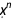of our fit. In the following code, I compute the MarginalDistribution of each component of the regression coefficient distributions and visualize their credible intervals: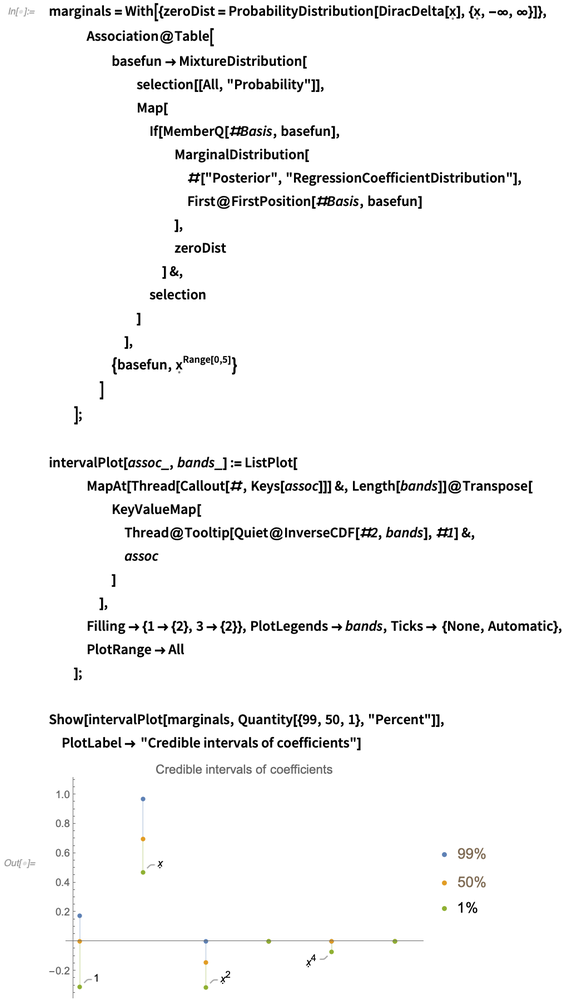✕ ```marginals = With[{zeroDist = ProbabilityDistribution[ DiracDelta[\[FormalX]], {\[FormalX], -\[Infinity], \ \[Infinity]}]}, Association@Table[ basefun -> MixtureDistribution[ selection[[All, "Probability"]], Map[ If[MemberQ[#Basis, basefun], MarginalDistribution[ #["Posterior", "RegressionCoefficientDistribution"], First@FirstPosition[#Basis, basefun] ], zeroDist ] &, selection ] ], {basefun, \[FormalX]^Range[0, 5]} ] ]; intervalPlot[assoc_, bands_] := ListPlot[ MapAt[Thread[Callout[#, Keys[assoc]]] &, Length[bands]]@Transpose[ KeyValueMap[ Thread@Tooltip[Quiet@InverseCDF[#2, bands], #1] &, assoc ] ], Filling -> {1 -> {2}, 3 -> {2}}, PlotLegends -> bands, Ticks -> {None, Automatic}, PlotRange -> All ]; Show[intervalPlot[marginals, Quantity[{99, 50, 1}, "Percent"]], PlotLabel -> "Credible intervals of coefficients"]```

Compare this against the credible intervals you get when you fit a single fifth-degree polynomial rather than 63 different ones, which is significantly less informative: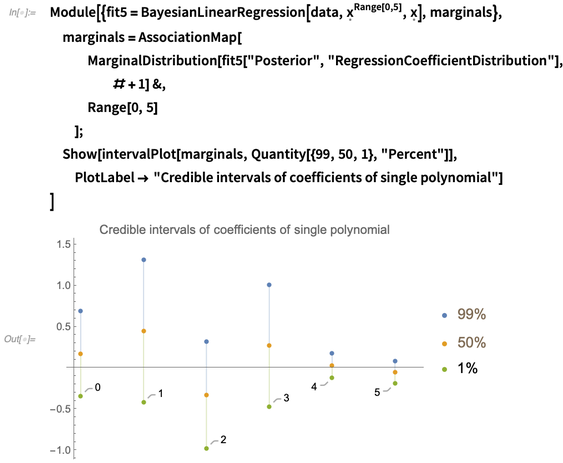✕ ```Module[{fit5 = BayesianLinearRegression[data, \[FormalX]^Range[0, 5], \[FormalX]], marginals}, marginals = AssociationMap[ MarginalDistribution[ fit5["Posterior", "RegressionCoefficientDistribution"], # + 1] &, Range[0, 5] ]; Show[intervalPlot[marginals, Quantity[{99, 50, 1}, "Percent"]], PlotLabel -> "Credible intervals of coefficients of single polynomial"] ]```

The first plot shows us we can be quite sure that the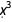and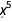terms in the polynomial are 0, and we now also know the signs of the,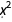and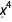terms, but we can only come to these conclusions by trying out all possible polynomials and comparing them against each other.

This procedure is also quite different from another popular method for making fitting terms disappear, which is called LASSO FitRegularization: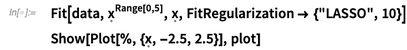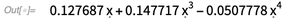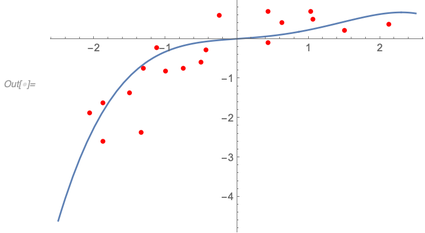✕ ```Fit[data, \[FormalX]^Range[0, 5], \[FormalX], FitRegularization -> {"LASSO", 10}] Show[Plot[%, {\[FormalX], -2.5, 2.5}], plot]```

Interestingly, the LASSO penalty used here discards the quadratic term rather than the cubic one, but the result depends on the strength of the regularization strength (which I somewhat arbitrarily set to 10). The main difference between these two procedures is that at no point during the Bayesian analysis did I have to apply an artificial penalty for models with more terms: it is a result that simply drops out after considering all the possibilities and working out their probabilities.